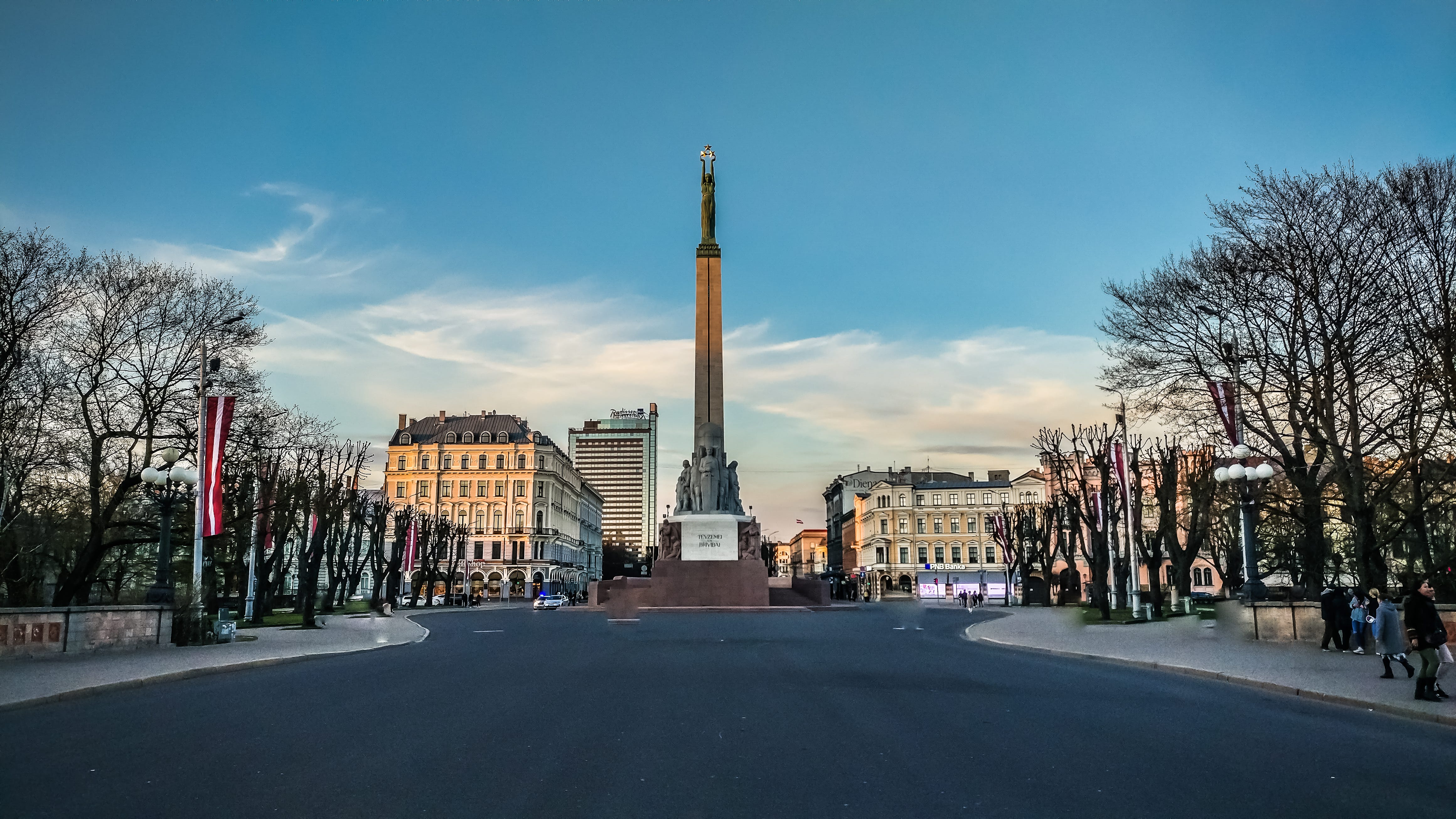# A Thought Experiment for Defining “Concept”

What is the concept? How do we define it? How our consciousness perceive, record, and recall a concept? In this post, I will try to answer these questions.

Yesterday, I went out. I just wanted to go out in the fresh-cold streets of Riga. It is snow everywhere, therefore I decided to walk instead of biking. First, I walk down through Birznieka Upisa Street. When I reach the entrance of the bazaar, I turned right and got inside of it. When I reached Knockout barbershop, I turned left and got out of the bazaar from the parking area. I walked through the Dzirnavu street and reached to Berga bazaar. I walked through the Berga bazaar and got out near a park that I still don’t know the name of. I walked and passed the park and reach the bus stop. I walked through the side street and reach Copy Pro. I passed the street and reach the monument of freedom.Photo of the monument of freedom by Priyank P on Unsplash

You may be asking me: “Why did you tell me all these now?”. I just defined you the concept of the small trip of mine. This is the main trip concept in my brain. If I would choose another street instead of passing through the bazaars, if I would take another path in the park while I was passing it, if I would start the trip from Lacplesa street, it would be another trip. So, what made this trip the specific concept that I just told you are the destinations I reached and the paths I took. One different path, one different intermediate destination would change the whole concept. Now, let’s define it with math. Trust me, it will be easier to understand if you haven’t so far.

Imagine you are on a point “.” in space (P1). the paths you take are vectors “ — — >”. After a vector(path)(V1) is applied to the point, another point is reached(P2). Then, another vector V2 is applied, and the point P3 is reached. On another time, you again start from P1 and take paths V3 and V4 which are different than V1 and V2. But you reach the same P3 at the end. IF the first trip is T1, and the second trip is T2, is t1 equals to T2?

If you say yes, it means that you actually care about the points you reached. The equation is not “T1 = T2” for you anymore. It is “P3 = P3”.

If you say no, it means that the paths are also important. The way you reach P3 also defines the trips T1 and T2.

If we assume the first statement which looks at the final destination to define the trips, each concept is actually a point in multi-dimensional space (In math, the point is basically a vector that defines that point. But since we used the vector to define a path, I will call it “point” instead of “vector”). In this case, the number of concepts that we can define is bounded to the dimension of space that we work on. If it is a human brain and the number of neurons is limited, it means that the number of concepts that we can learn is limited. But, is it so? I do not know. What I know is that we are capable of learning a pretty high number of concepts.

If we assume the second statement which looks at the paths as well as intermediate and final destinations, each concept is the overall path that we take. Therefore, if the starting points are the same, the vectors(paths) are the same and the queue of applied vectors is the same (when to take each path), then the concepts are the same. In this case, the number of concepts that can be defined is not limited to destination points, because it also depends on the paths and when to take each path. If Riga is my brain, a concept is not only the monument of freedom but also the way I went there. If this is the case, then we should look not only at the specific neurons which are activated in the brain when a concept is learned, we also should look at the neurons that activate those specific neurons and also the time(the queue) that they activate those neurons. We basically need to trace an activated neuron path to monitor the recording and recall of a concept in the brain.

Let’s think about the from the artificial neural networks side. Let’s assume that we have fully connected layers in an ANN. The output represents the concepts that the ANN learns. Let’s assume that the 3rd neuron in the output layer is activated with an input. Normally, we say that the neuron that is activated at the output layer represents the concept of the input. This statement is accepted and it actually represents the first statement that I mentioned above (if destination points are the same, then the concepts are the same). What if the second statement is applied to the ANNs? In this case, the activation path in the ANN defines the concept. Currently, I do not know how to build this mathematically so that I can code it to try it out. But the next post will be about this.

If you would like to share your idea on this, do not hesitate to comment below. Ideas merge and get more valuable when they are shared.

## More from Tekin Evrim Ozmermer

AI Developer In “>”, Interested in Artificial Intelligence, Human Intelligence, Economical inequality, and all other interesting stuff.